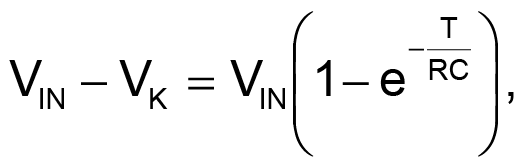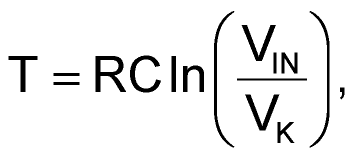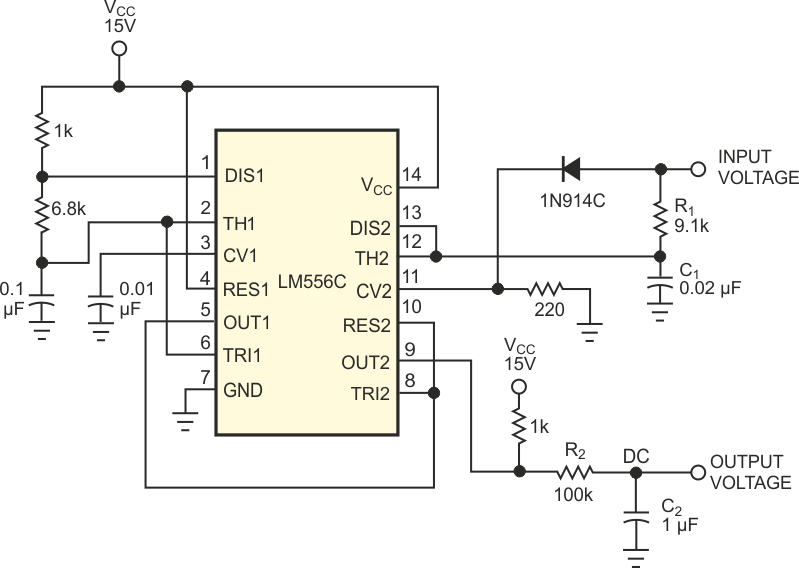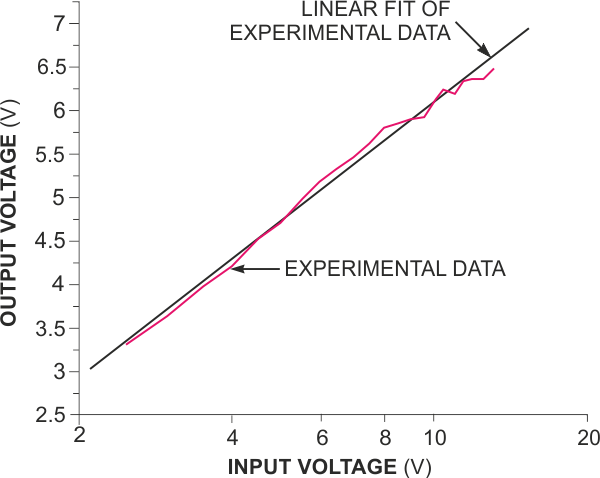# Log amp uses capacitor-charging law

## Texas Instruments LM556

Jayashree Raghuraman

EDN

The novel logarithmic amplifier in Figure 1 relies on the exponential charging characteristics of a simple RC circuit. The expression for the time, T, required for a capacitor, C, to reach a voltage (VIN–VK) from 0 V, when charged through a resistor, R, with an applied voltage of VIN, is(1)

where VK is a fixed voltage. The expression for T reduces to(2)

clearly showing an inherent logarithmic characteristic. The circuit in Figure 1 demonstrates this characteristic, using a 556 timer. With the values shown, the first stage of the 556 timer is a standard astable circuit operating at a frequency of approximately 1 kHz. The output of this stage acts as the trigger for the second stage. The second stage operates as a modified monostable circuit. In this modified configuration, the RC combination, R1 and C1, charges from an external voltage, VIN, instead of VCC. The control-voltage pin, CV2, has the value VIN minus one diode drop, VK.Figure 1. A simple 556 timer depends on RC charging to form a logarithmic amplifier.

Table 1. Output versus input voltage
 Input voltage Output voltage Input voltage Output voltage 2.5 3.324 8 5.782 3 3.667 8.5 5.861 3.5 3.954 9 5.886 4 4.227 9.5 5.945 4.5 4.506 10 6.098 5 4.705 10.5 6.187 5.5 4.956 11 6.204 6 5.151 11.5 6.312 6.5 5.315 12 6.371 7 5.444 12.5 6.378 7.5 5.615 13 6.476

The monostable pulse width, T, then depends on the time required for capacitor C1 to charge to VIN–VK through R1 with the applied voltage VIN. The output of the second stage, filtered through R2 and C2, depends on the first stage's astable frequency; the supply voltage, VCC; and the monostable pulse width, T. Because VCC and the astable frequency are constant, VOUT is proportional to T. Table 1 tabulates the experimental results, and Figure 2 shows graphical results. The circuit operation is limited to an input range of 2.5 to 13 V to satisfy the internal biasing requirements of the second stage of the 556. The diode drop, VK, is not strictly constant, because it varies with current. In spite of these limitations, Table 1 and Figure 2 clearly show a distinct logarithmic characteristic.Figure 2. The circuit of Figure 1 produces a distinct logarithmic output.

EDN